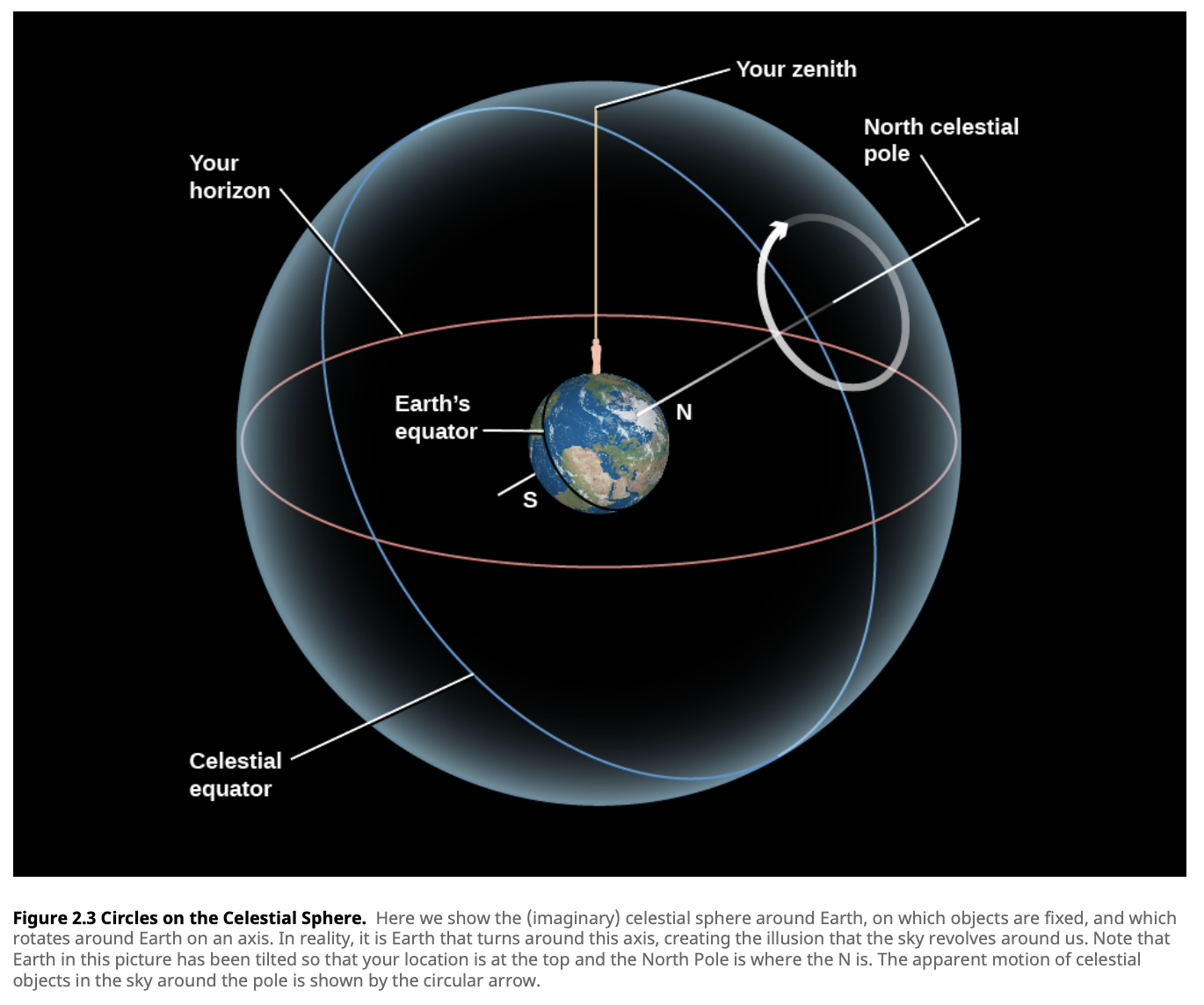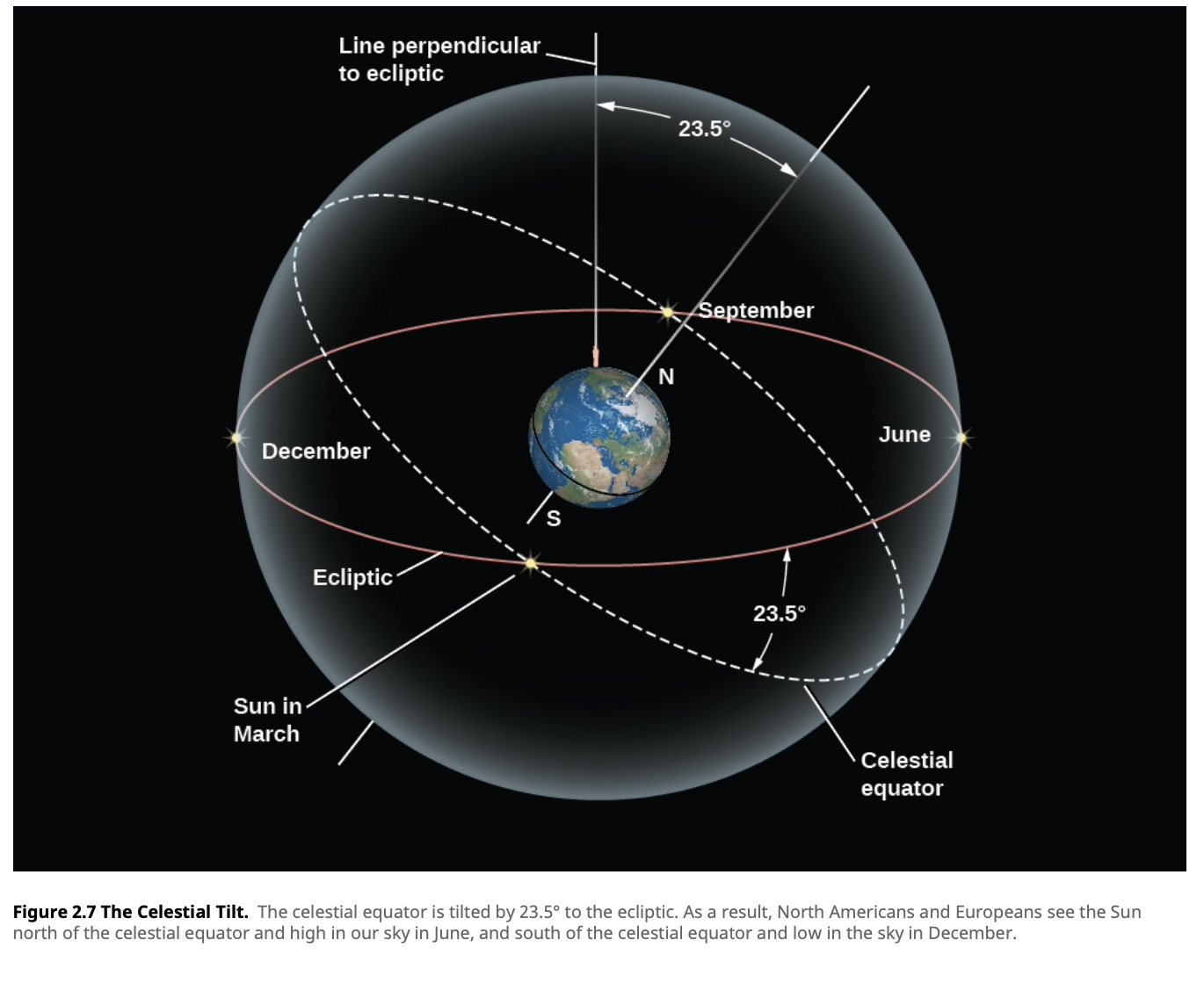# Celestial Sphere• An imaginary, hollow dome surrounding the Earth
• It's essentially the sky we see when we look up
• Earth's coordinate system has been extended to the sphere
• It's what you see when you look up at the sky; you are at the center
• The path the Sun takes on the celestial sphere is called the ecliptic
• Apparent yearly path of the Sun
• Projection of the Earth's orbit onto the sky
• Tilted by 23.5 degrees with respect to the celestial equator## Coordinate System

• Declination
• Vertical position of an object
• Celestial equivalent of latitude
• Degrees, arcmin, arcsec
• Right ascension
• Horizontal position of an object
• Celestial equivalent of longitude
• Expressed as either degrees or units of time
• Celestial sphere turns around the Earth once a day
• As Earth turns on its axis
• 360˚ can easily just be 24 hours
• 15˚ arc is equal to 1 hour
• Hours, mins, sec
• Starts getting counted at the vernal equinox
• Altitude
• Vertical position of an object
• Angle from the horizon to a point on the sphere
• Zenith is 90˚
• Altitude of Polaris with give you the latitude of where you are on Earth
• Azimuth
• Horizontal position of an object
• Angle measured clockwise around observer's horizon from north
• Object due north has azimuth of 0˚

• Zenith